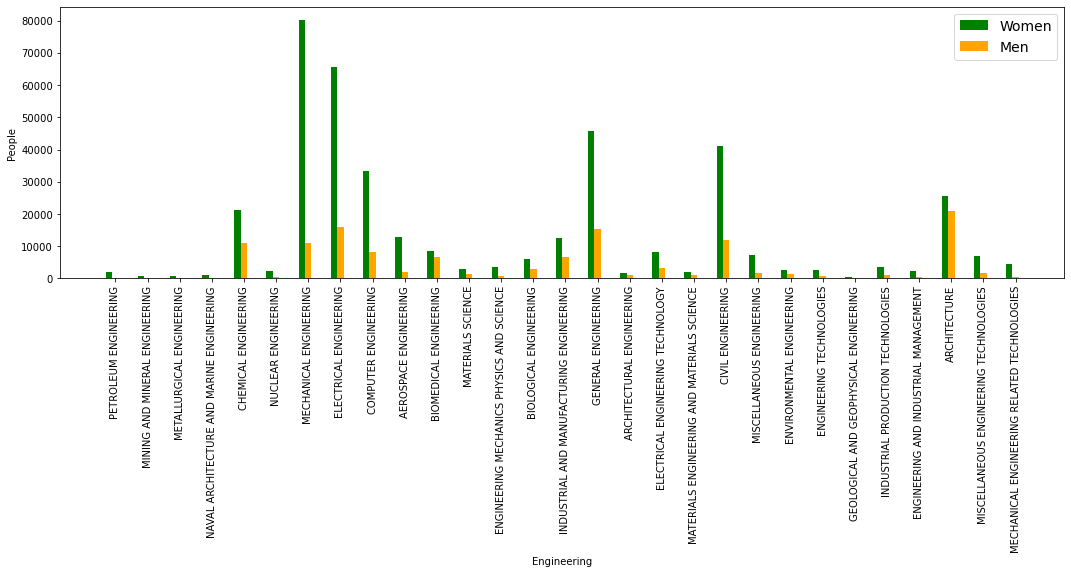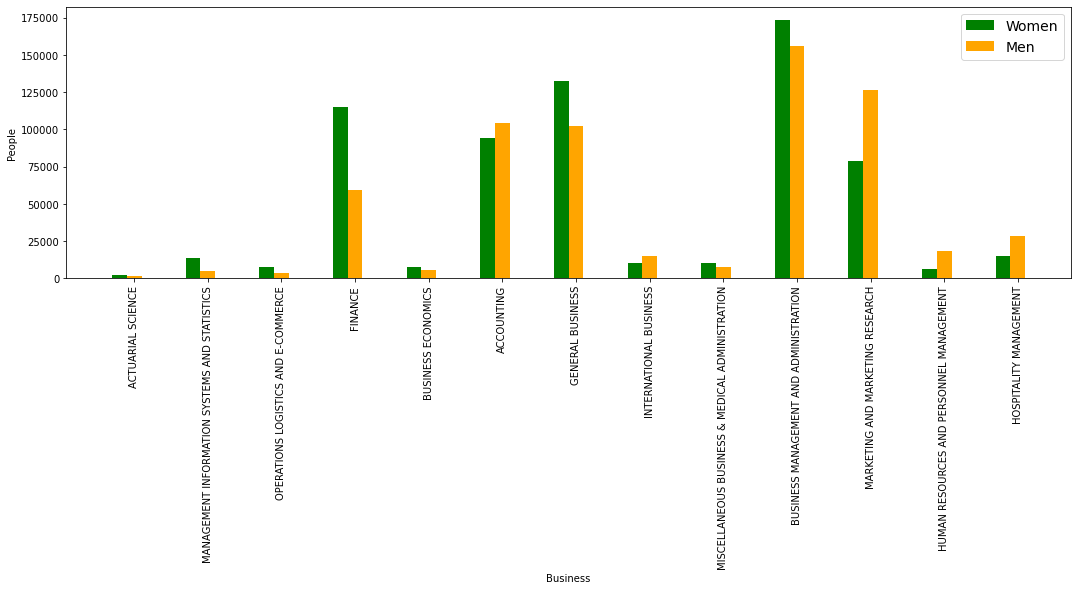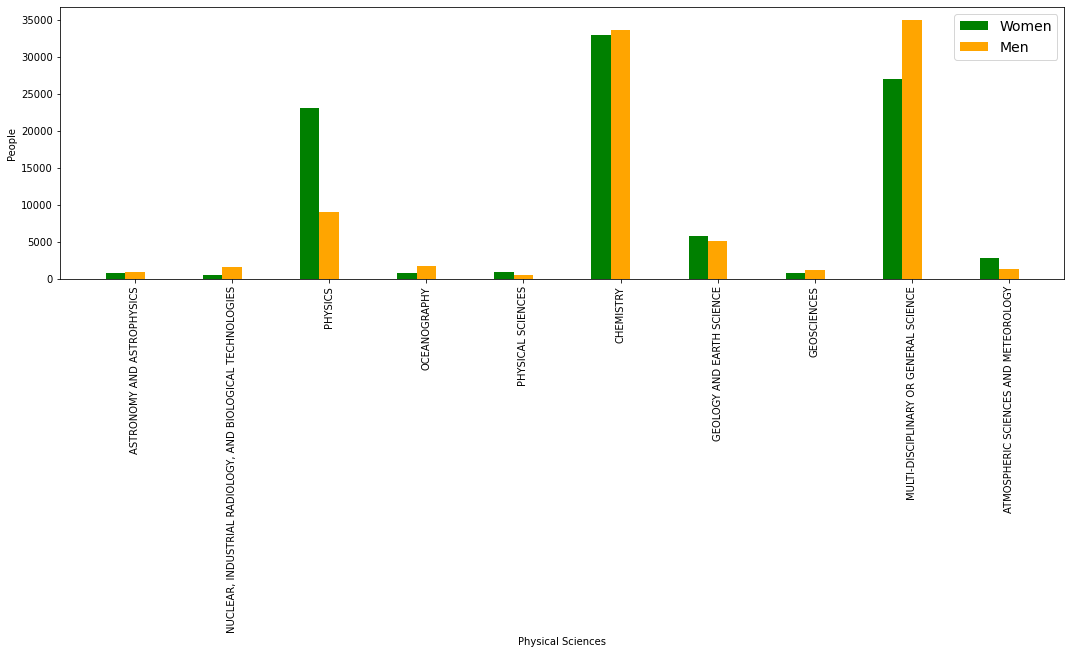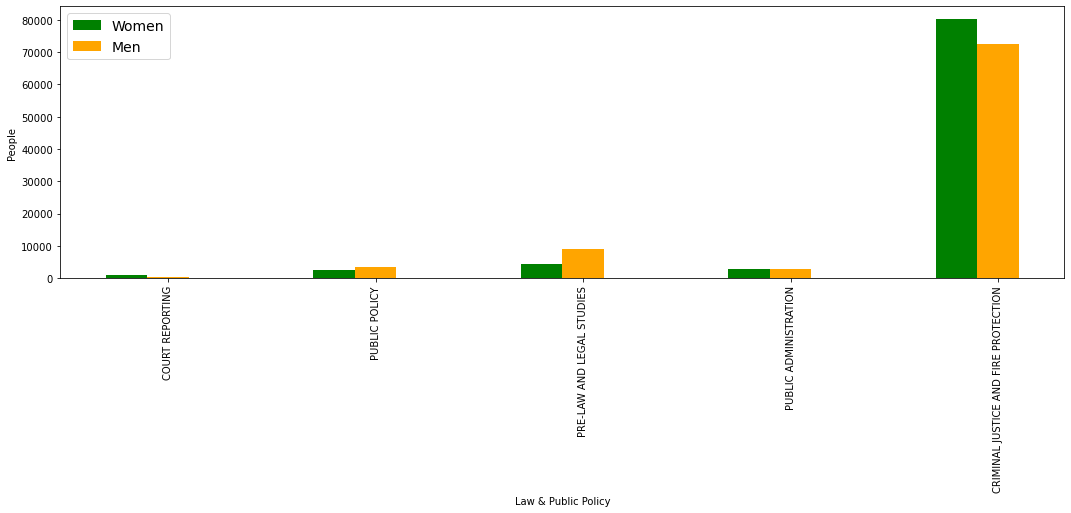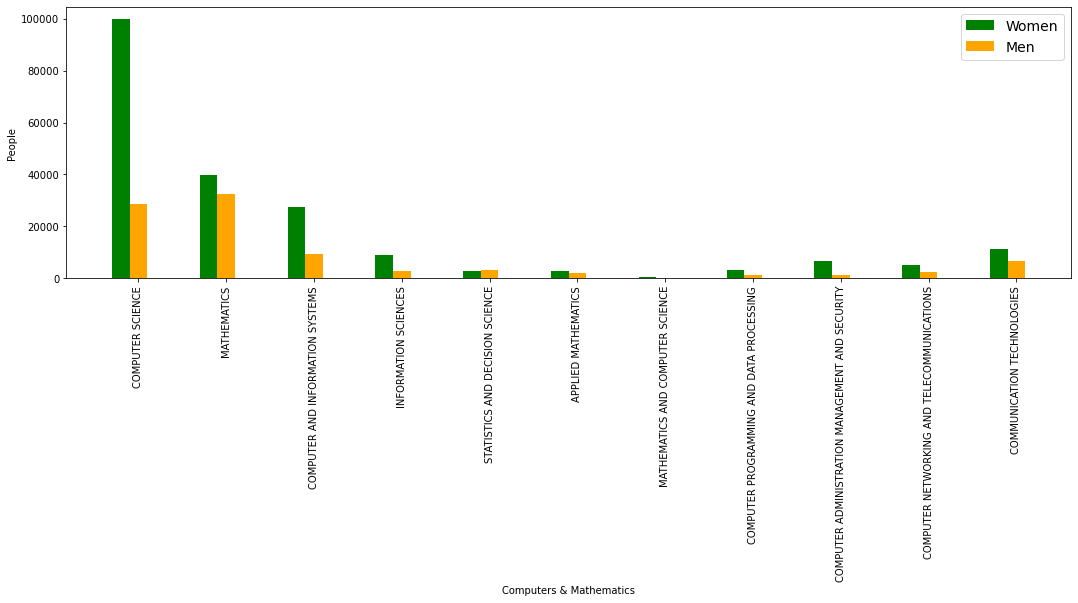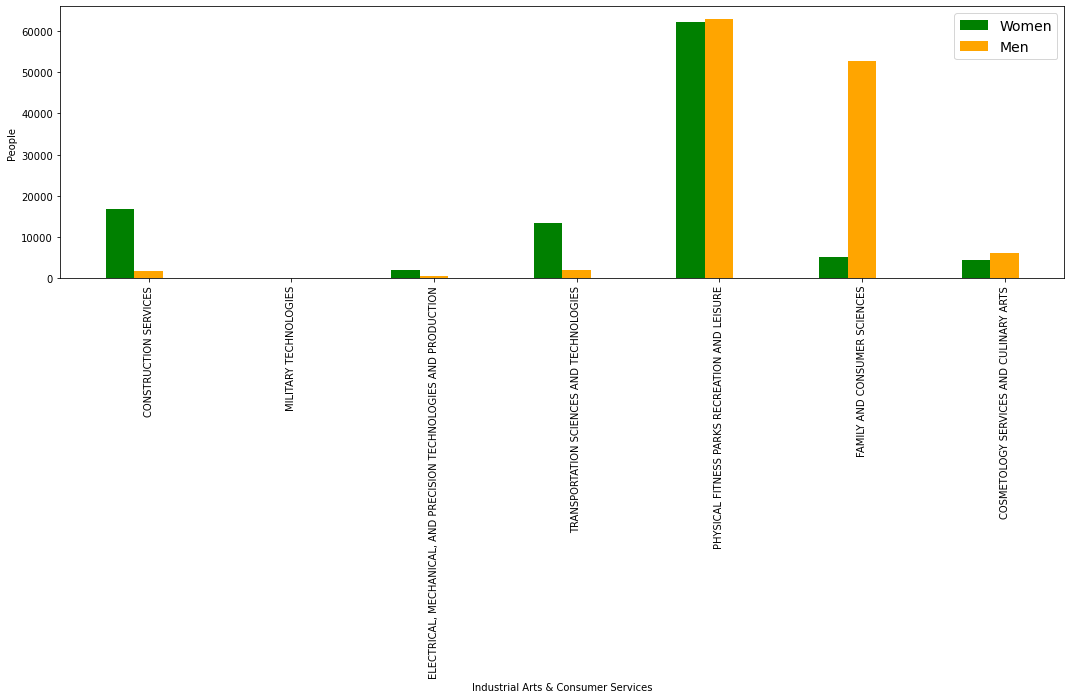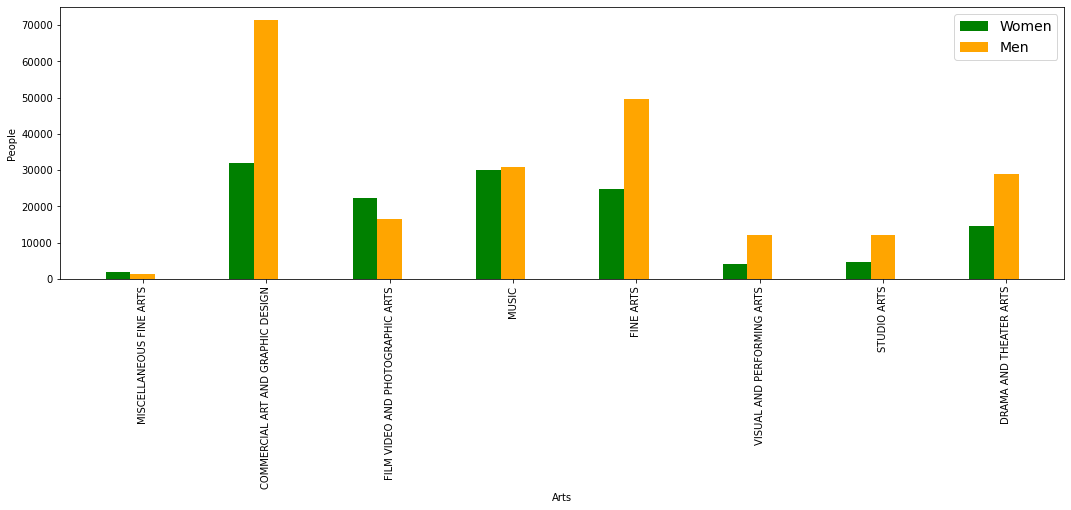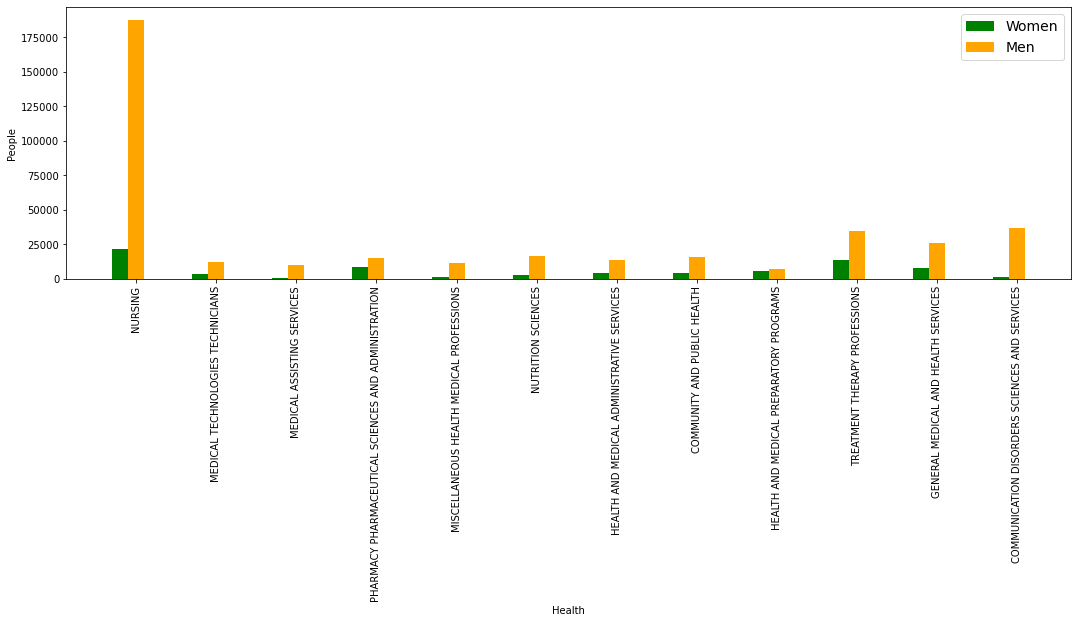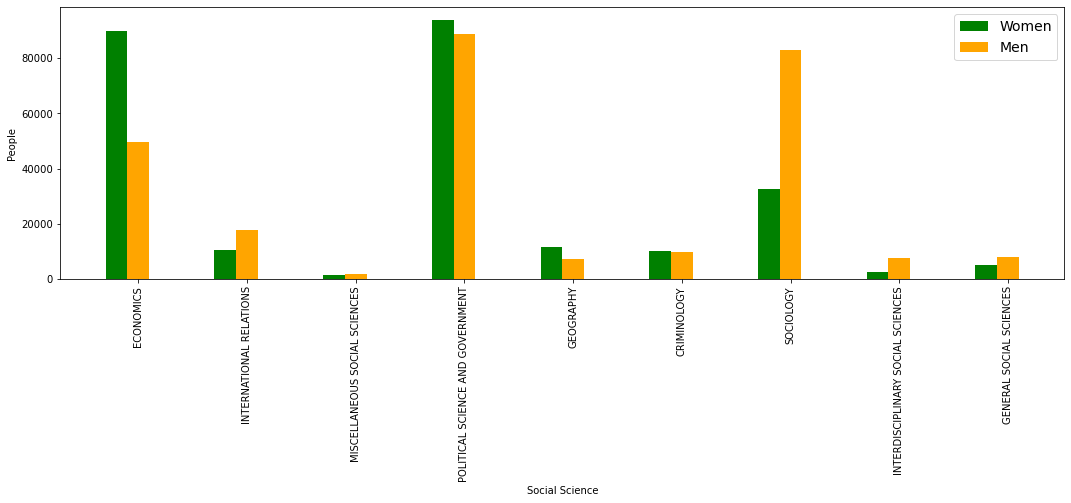# Guided Project:¶

## Visualizing Earnings Based On College Majors¶

In this course, we've been creating plots using pyplot and matplotlib directly.

When we want to explore a new dataset by quickly creating visualizations, using these tools directly can be cumbersome.

Thankfully, pandas has many methods for quickly generating common plots from data in DataFrames. Like pyplot, the plotting functionality in pandas is a wrapper for matplotlib.

This means we can customize the plots when necessary by accessing the underlying Figure, Axes, and other matplotlib objects.

In this guided project, we'll explore how using the pandas plotting functionality along with the Jupyter notebook interface allows us to explore data quickly using visualizations.

Trabajaremos con un conjunto de datos sobre:

• We'll be working with a dataset on the job outcomes of students who graduated from college between 2010 and 2012.

The original data on job outcomes was released by American Community Survey, which conducts surveys and aggregates the data. FiveThirtyEight cleaned the dataset and released it on their Github repo.

Each row in the dataset represents a different major in college and contains information on:

• Gender diversity.

• Employment rates.

• Median salaries, and more.

Here are some of the columns in the dataset:

• Rank - Rank by median earnings (the dataset is ordered by this column).

• Major_code - Major code.

• Major - Major description.

• Major_category - Category of major.

• Total - Total number of people with major.

• Sample_size - Sample size (unweighted) of full-time.

• Men - Male graduates.

• Women - Female graduates.

• ShareWomen - Women as share of total.

• Employed - Number employed.

• Unemployment_rate - Percentage of the work force that is unemployed at any given date

• Median - Median salary of full-time, year-round workers.

• Low_wage_jobs - Number in low-wage service jobs.

• Full_time - Number employed 35 hours or more.

• Part_time - Number employed less than 35 hours.

Using visualizations, we can start to explore questions from the dataset like:

• Do students in more popular majors make more money?

• Using scatter plots
• How many majors are predominantly male? Predominantly female?

• Using histograms
• Which category of majors have the most students?

• Using bar plots

Nota: La relación entre el tipo de visualización, está relacionada con la pregunta, por ejemplo:

• Cuando se hace referencia a los estudiantes (a un grupo en general) se usa scatter plot.

• Cuando se quiere separar por genero entonces se usan histogramas.

• Cuando se habla de categorias entonces los graficos de barras.

We'll explore how to do these and more while primarily working in pandas. Before we start creating data visualizations, let's import the libraries we need and remove rows containing null values.

• Let's setup the environment by importing the libraries we need and running the necessary Jupyter magic %matplotlib inline so that plots are displayed inline.
In :
import matplotlib.pyplot as plt
import pandas as pd
import numpy as np

In :
recent_grads = pd.read_csv("recent-grads.csv")


Using DataFrame.iloc[] to return the first row formatted as a table.

In :
recent_grads.iloc[:1]

Out:
Rank Major_code Major Total Men Women Major_category ShareWomen Sample_size Employed ... Part_time Full_time_year_round Unemployed Unemployment_rate Median P25th P75th College_jobs Non_college_jobs Low_wage_jobs
0 1 2419 PETROLEUM ENGINEERING 2339.0 2057.0 282.0 Engineering 0.120564 36 1976 ... 270 1207 37 0.018381 110000 95000 125000 1534 364 193

1 rows × 21 columns

• Use of DataFrame.head() and DataFrame.tail() to see how the data is structured.
In :
recent_grads.head(3)

Out:
Rank Major_code Major Total Men Women Major_category ShareWomen Sample_size Employed ... Part_time Full_time_year_round Unemployed Unemployment_rate Median P25th P75th College_jobs Non_college_jobs Low_wage_jobs
0 1 2419 PETROLEUM ENGINEERING 2339.0 2057.0 282.0 Engineering 0.120564 36 1976 ... 270 1207 37 0.018381 110000 95000 125000 1534 364 193
1 2 2416 MINING AND MINERAL ENGINEERING 756.0 679.0 77.0 Engineering 0.101852 7 640 ... 170 388 85 0.117241 75000 55000 90000 350 257 50
2 3 2415 METALLURGICAL ENGINEERING 856.0 725.0 131.0 Engineering 0.153037 3 648 ... 133 340 16 0.024096 73000 50000 105000 456 176 0

3 rows × 21 columns

In :
recent_grads.tail(3)

Out:
Rank Major_code Major Total Men Women Major_category ShareWomen Sample_size Employed ... Part_time Full_time_year_round Unemployed Unemployment_rate Median P25th P75th College_jobs Non_college_jobs Low_wage_jobs
170 171 5202 CLINICAL PSYCHOLOGY 2838.0 568.0 2270.0 Psychology & Social Work 0.799859 13 2101 ... 648 1293 368 0.149048 25000 25000 40000 986 870 622
171 172 5203 COUNSELING PSYCHOLOGY 4626.0 931.0 3695.0 Psychology & Social Work 0.798746 21 3777 ... 965 2738 214 0.053621 23400 19200 26000 2403 1245 308
172 173 3501 LIBRARY SCIENCE 1098.0 134.0 964.0 Education 0.877960 2 742 ... 237 410 87 0.104946 22000 20000 22000 288 338 192

3 rows × 21 columns

• Generate summary statistics for all of the numeric columns using DataFrame.describe().
In :
recent_grads.describe()

Out:
Rank Major_code Total Men Women ShareWomen Sample_size Employed Full_time Part_time Full_time_year_round Unemployed Unemployment_rate Median P25th P75th College_jobs Non_college_jobs Low_wage_jobs
count 173.000000 173.000000 172.000000 172.000000 172.000000 172.000000 173.000000 173.000000 173.000000 173.000000 173.000000 173.000000 173.000000 173.000000 173.000000 173.000000 173.000000 173.000000 173.000000
mean 87.000000 3879.815029 39370.081395 16723.406977 22646.674419 0.522223 356.080925 31192.763006 26029.306358 8832.398844 19694.427746 2416.329480 0.068191 40151.445087 29501.445087 51494.219653 12322.635838 13284.497110 3859.017341
std 50.084928 1687.753140 63483.491009 28122.433474 41057.330740 0.231205 618.361022 50675.002241 42869.655092 14648.179473 33160.941514 4112.803148 0.030331 11470.181802 9166.005235 14906.279740 21299.868863 23789.655363 6944.998579
min 1.000000 1100.000000 124.000000 119.000000 0.000000 0.000000 2.000000 0.000000 111.000000 0.000000 111.000000 0.000000 0.000000 22000.000000 18500.000000 22000.000000 0.000000 0.000000 0.000000
25% 44.000000 2403.000000 4549.750000 2177.500000 1778.250000 0.336026 39.000000 3608.000000 3154.000000 1030.000000 2453.000000 304.000000 0.050306 33000.000000 24000.000000 42000.000000 1675.000000 1591.000000 340.000000
50% 87.000000 3608.000000 15104.000000 5434.000000 8386.500000 0.534024 130.000000 11797.000000 10048.000000 3299.000000 7413.000000 893.000000 0.067961 36000.000000 27000.000000 47000.000000 4390.000000 4595.000000 1231.000000
75% 130.000000 5503.000000 38909.750000 14631.000000 22553.750000 0.703299 338.000000 31433.000000 25147.000000 9948.000000 16891.000000 2393.000000 0.087557 45000.000000 33000.000000 60000.000000 14444.000000 11783.000000 3466.000000
max 173.000000 6403.000000 393735.000000 173809.000000 307087.000000 0.968954 4212.000000 307933.000000 251540.000000 115172.000000 199897.000000 28169.000000 0.177226 110000.000000 95000.000000 125000.000000 151643.000000 148395.000000 48207.000000

It can be seen that there are columns that have different lengths.

Therefore I will start droping all rows with missing values.

Matplotlib expects that columns of values we pass in have matching lengths and missing values will cause matplotlib to throw errors.

Look up the number of rows in recent_grads and assign the value to raw_data_count.

In :
raw_data_count = len(recent_grads)
raw_data_count

Out:
173

By using DataFrame.dropna() for drop rows containing missing values and assign the resulting DataFrame back to recent_grads.

In :
recent_grads.dropna( axis=0, inplace=True)

In :
cleaned_data_count = len(recent_grads)
cleaned_data_count

Out:
172

Look up the number of rows in recent_grads now and assign the value to cleaned_data_count.

If you compare cleaned_data_count and raw_data_count, you'll notice that only one row contained missing values and was dropped.

There was only one line that could not be counted on;

$173 -172 = 1$

## 2. Pandas and the Scatter Plots¶

Most of the plotting functionality in pandas is contained within the DataFrame.plot() method.

When we call this method, we specify the data we want plotted as well as the type of plot.

We use the kind parameter to specify the type of plot we want. We use x and y to specify the data we want on each axis. You can read about the different parameters in the documentation.

### kind types¶

• ‘line’ : line plot (default)

• ‘bar’ : vertical bar plot

• ‘barh’ : horizontal bar plot

• ‘hist’ : histogram

• ‘box’ : boxplot

• ‘kde’ : Kernel Density Estimation plot

• ‘density’ : same as ‘kde’

• ‘area’ : area plot

• ‘pie’ : pie plot

• ‘scatter’ : scatter plot (DataFrame only)

• ‘hexbin’ : hexbin plot (DataFrame only)

### The same graph through different types of kind.¶

By way of learning I find interesting to show the different types of graphs that can be represented with the variations of kind parameter.

In this case it is not important if the visualization is adequate or not, what is intended to show is the different representations offered by the kind parameter.

In :
kind_types = ['line', 'bar','barh', 'hist', 'box', 'kde', 'density', 'area', 'pie', 'scatter', 'hexbin']

for i in kind_types:
recent_grads.plot(x='Sample_size', y='Employed', kind = i )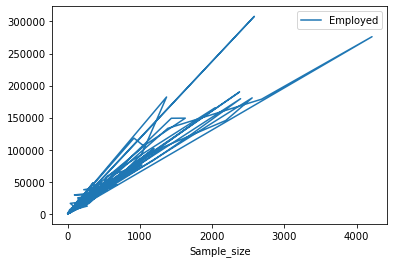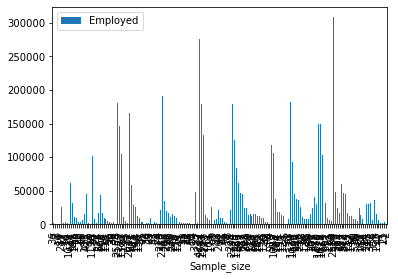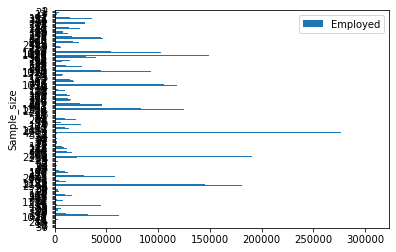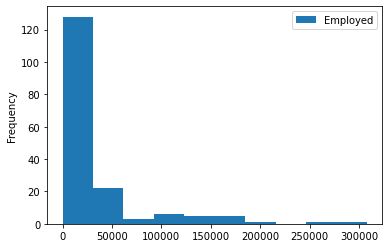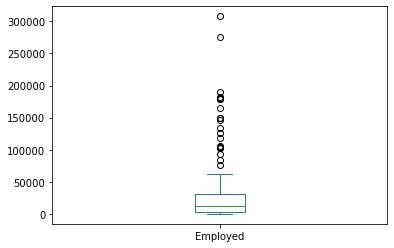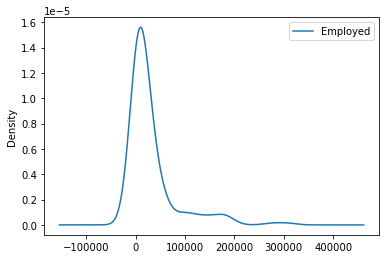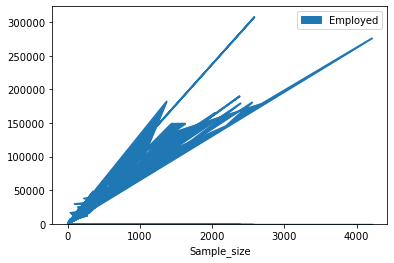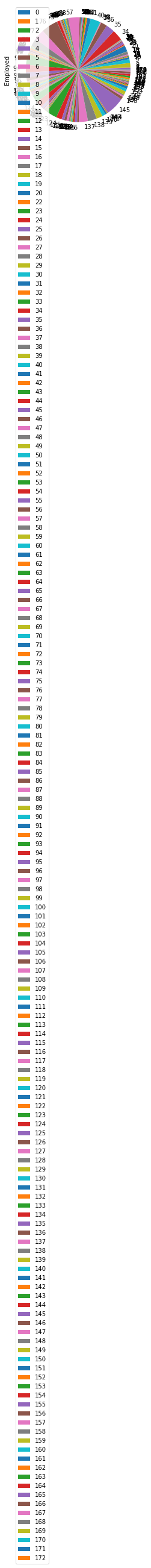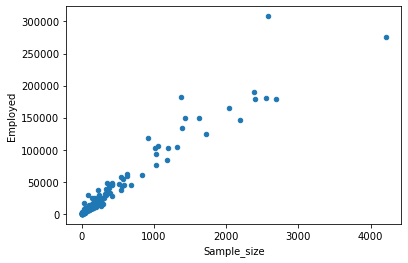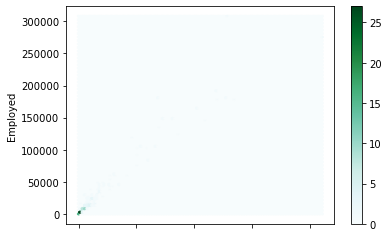### Note about using DataFrame.plot()¶

The DataFrame.plot() method has a few parameters we can use for tweaking the scatter plot:

• x =
• y =
• kind =
• title =
• figsize =

But we can instantiate an object and make use of the methods that correspond to that object.

In :
ax = recent_grads.plot(x='Sample_size', y='Employed', kind='scatter')
ax.set_title('Employed vs. Sample_size')

Out:
Text(0.5, 1.0, 'Employed vs. Sample_size')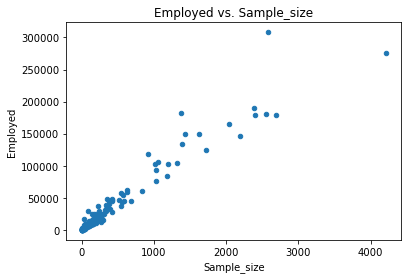• It can be said that there is a correlation between the measurement of the samples and the number of employees

• To explore the following relations generate a series of scatter plots :

### - Sample_size vs. Median¶

• Sample_size - Sample size (unweighted) of full-time.

• Median - Median salary of full-time, year-round workers.

In :
recent_grads.plot(x='Sample_size',
y='Median',
kind='scatter',

title='Sample size of full-time vs. median salary of full-time, year-round workers.')

Out:
<AxesSubplot:title={'center':'Sample size of full-time vs. median salary of full-time, year-round workers.'}, xlabel='Sample_size', ylabel='Median'>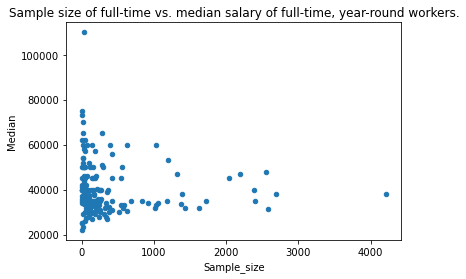A small number of full-time samples have the highest range of salaries. on the contrary, when the samples are larger, the salary decreases.

### - Sample_size vs. Unemployment_rate¶

• Sample_size - Sample size (unweighted) of full-time.

• Unemployment_rate - The percentage of the work force that is unemployed at any given date

In :
recent_grads.plot(x='Sample_size',
y='Unemployment_rate',
kind='scatter',
title='Sample_size vs. Unemployment_rate')

Out:
<AxesSubplot:title={'center':'Sample_size vs. Unemployment_rate'}, xlabel='Sample_size', ylabel='Unemployment_rate'>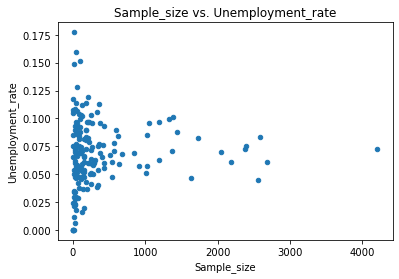Similarly when the samples are small the unemployment relationship covers a fairly wide range, however when the size of the full-time samples increases the unemployment ratio is focused on central elements of our graph.

### - Full_time vs. Median¶

• Full_time - Number employed 35 hours or more.

• Median - Median salary of full-time, year-round workers.

In :
recent_grads.plot(x='Full_time',
y='Median',
kind='scatter',
title='Full_time vs. Median')

Out:
<AxesSubplot:title={'center':'Full_time vs. Median'}, xlabel='Full_time', ylabel='Median'>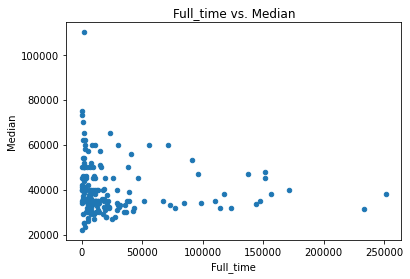This graph explains how a minority of people between 0 and 2500 approximately taking into account that the scale fund with which we are working is 250000, work more than 35 hours and the average salary they have goes from 20000 to 80000 by the way as the number of people who work full time increases the salary has a tendency to decrease.

### - ShareWomen vs. Unemployment_rate¶

• ShareWomen - Women as share of total.

• Unemployment_rate - The percentage of the work force that is unemployed at any given date.

In :
recent_grads.plot(x='ShareWomen',
y='Unemployment_rate',
kind='scatter',
title='ShareWomen vs. Unemployment_rate')

Out:
<AxesSubplot:title={'center':'ShareWomen vs. Unemployment_rate'}, xlabel='ShareWomen', ylabel='Unemployment_rate'>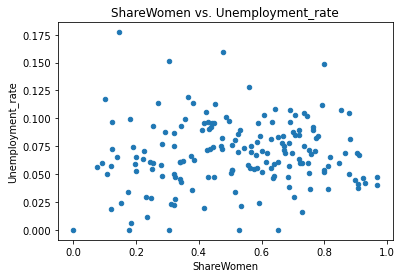It can be observed that the unemployment relationship among women is distributed in a fairly broad way within this group.

### - Men vs. Median¶

• Men - Men.

• Unemployment_rate Unemployment rate

In :
recent_grads.plot(x='Men',
y='Median',
kind='scatter',

title='Men vs. Median')

Out:
<AxesSubplot:title={'center':'Men vs. Median'}, xlabel='Men', ylabel='Median'>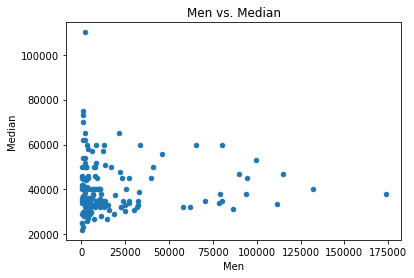as we can see there is a large minority of men who earn an average salary of between 20000 and 80000 and that as that number of men increases the salary also decreases

### - Women vs Median¶

• Women - Women.

• Median - Median salary of full-time, year-round workers.

In :
recent_grads.plot(x='Women',
y='Median',
kind='scatter',
title='Women vs. Median')

Out:
<AxesSubplot:title={'center':'Women vs. Median'}, xlabel='Women', ylabel='Median'>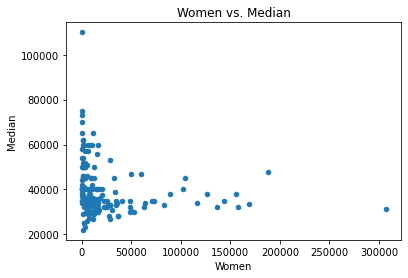• the same trend occurs in the graphics of women, however we must bear in mind that the number of women here is greater with respect to the graphics of men and that will affect the visualization of our graphics.
• As a remarkable fact we can say that although the salaries at their top can be equivalent, the differences are found in that the average in men is even higher than in women.

## 2.1 Matplotlib, much more detailed plots.¶

Because the data that we are going to handle are not categorical, therefore they will be numerical, the ideal in this case is to use a histogram

a histogram is a graph through which it is possible to represent with rectangles distributions of frequencies on determined coordinates. That is, they let us know the frequency of a specific event, thanks to the distribution of information.

A way to do an exploratory analysis and observe the relationships of the data to be analyzed.

In :
cols = ["Sample_size",
"Median",
"Employed",
"Full_time", "ShareWomen", "Unemployment_rate", "Men", "Women"]

fig = plt.figure(figsize=(20,40))

r = 0

col_name = cols[r]
ax = recent_grads[col_name].plot(kind='hist', rot=0)
ax.set_xlabel(col_name, rotation = 0)

Out:
Text(0.5, 0, 'Sample_size')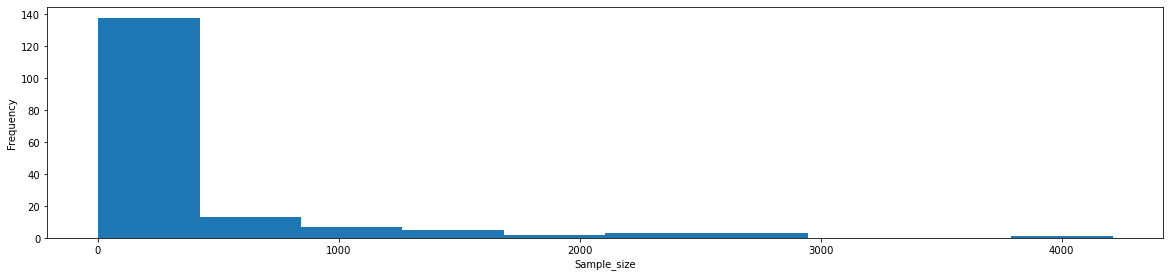the histogram informs us that the highest frequency of our full-time sample size set is the samples with a low value.

In :
cols = ["Sample_size",
"Median",
"Employed",
"Full_time", "ShareWomen", "Unemployment_rate", "Men", "Women"]

fig = plt.figure(figsize=(20,40))

r = 1

col_name = cols[r]
ax = recent_grads[col_name].plot(kind='hist', rot=0, bins = 50)
ax.set_xlabel(col_name, rotation = 0)

Out:
Text(0.5, 0, 'Median')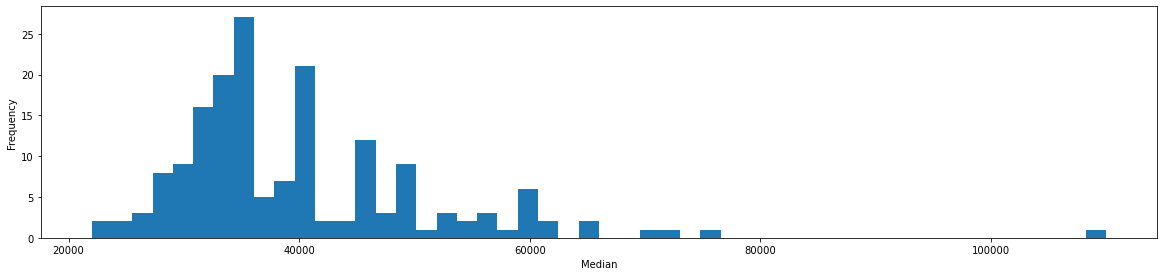In this case we see that the amount that is repeated more times (the frequency) of Median salary of full-time, year-round workers. is around 3000o dollars approximately, therefore students in more popular majors make more money.

In :
cols = ["Sample_size",
"Median",
"Employed",
"Full_time", "ShareWomen", "Unemployment_rate", "Men", "Women"]

fig = plt.figure(figsize=(20,40))

r = 2

col_name = cols[r]
ax = recent_grads[col_name].plot(kind='hist', rot=0, bins = 50)
ax.set_xlabel(col_name, rotation = 0)

Out:
Text(0.5, 0, 'Employed')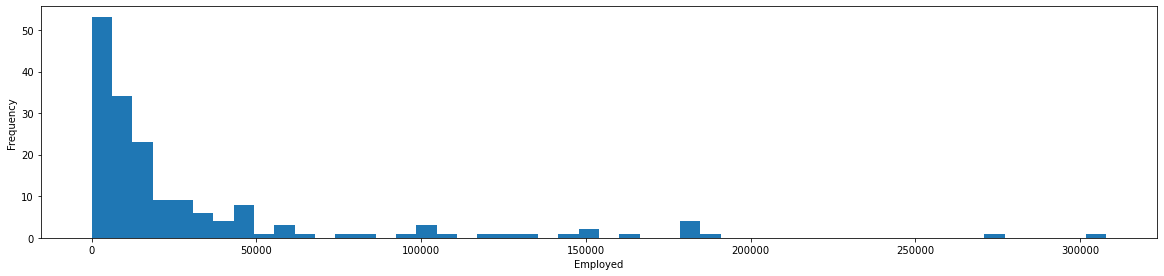In a skewed distribution, we see the following:

• In the case of the casual histogram, the values pile up toward the starting point of the range.

• Values decrease in frequency towards the opposite end, forming the tail of the distribution.

• Therefore the higher frequency of number of Employed is quite low.

In :
cols = ["Sample_size",
"Median",
"Employed",
"Full_time", "ShareWomen", "Unemployment_rate", "Men", "Women"]

fig = plt.figure(figsize=(20,40))

r = 3

col_name = cols[r]
ax = recent_grads[col_name].plot(kind='hist', rot=0, bins = 50)
ax.set_xlabel(col_name, rotation = 0)

Out:
Text(0.5, 0, 'Full_time')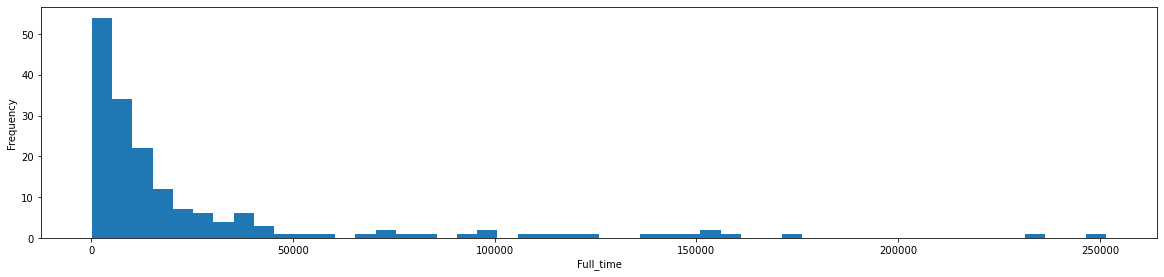The same goes for full-time jobs, the pattern of the graph above is repeated.

In :
cols = ["Sample_size",
"Median",
"Employed",
"Full_time", "ShareWomen", "Unemployment_rate", "Men", "Women"]

fig = plt.figure(figsize=(20,40))

r = 4

col_name = cols[r]
ax = recent_grads[col_name].plot(kind='hist', rot=0, bins = 50)
ax.set_xlabel(col_name, rotation = 0)

Out:
Text(0.5, 0, 'ShareWomen')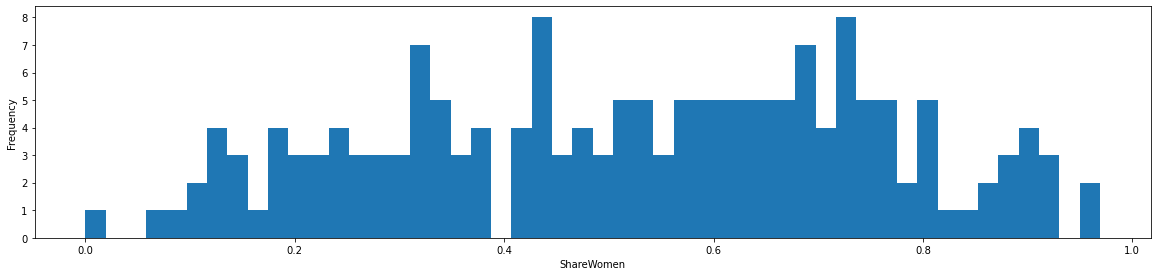We can see that ShareWomen distribution is homogeneous in the dataset.

In :
cols = ["Sample_size",
"Median",
"Employed",
"Full_time", "ShareWomen", "Unemployment_rate", "Men", "Women"]

fig = plt.figure(figsize=(20,40))

r = 5

col_name = cols[r]
ax = recent_grads[col_name].plot(kind='hist', rot=0, bins = 50)
ax.set_xlabel(col_name, rotation = 0)

Out:
Text(0.5, 0, 'Unemployment_rate')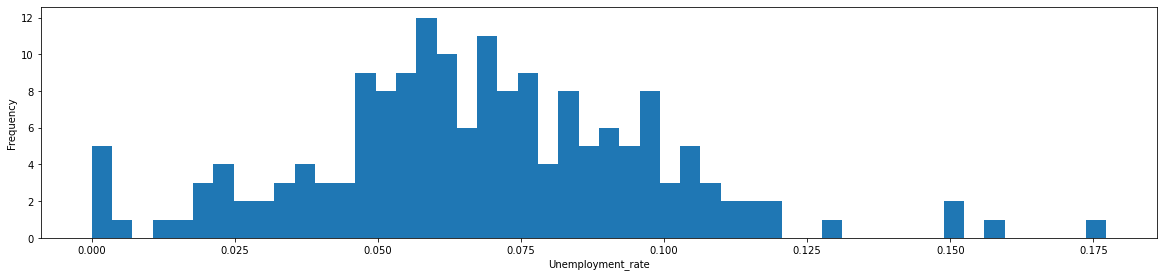The unemployment_rate can be said to be low since the values on the x-axis are low and the distribution covers a fairly small portion.

In :
plt.hist(recent_grads['Women'], label= "Women")

plt.legend()
plt.show()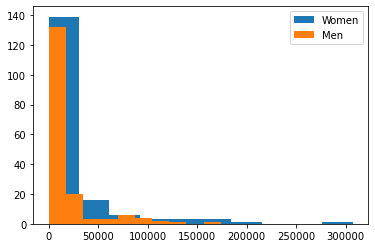Through the superposition of graphs we can observe that the total amount and frequency is greater for women.

In the graphs in which we compared the average salary between the two genders this we already saw in the x axis.

## - 2. How many majors are predominantly male?¶

The Mayors category type the most advisable thing would be to work instead of scatter plots with bar plots.

In :
plt.plot(recent_grads['Women'], label= "Women")

plt.legend()
plt.show()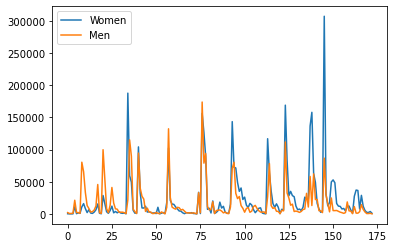Quickly we observe that women surpass men and that these are more focused on fields other than men.

In :
max_value = recent_grads["Men"].max()
max_value # max number of Men.

Out:
173809.0
In :
max_men_value_bool = recent_grads["Men"] == max_value
max_men_value_bool.unique()

Out:
array([False,  True])
In :
M_final_col = ["Major_category"]
M_final_col

Out:
['Major_category']

Through Boolean filtering we obtain which is the most chosen career category

In :
result = recent_grads.loc[max_men_value_bool,M_final_col]
result

Out:
Major_category

## ... and majors predominantly female?¶

In :
max_value = recent_grads["Women"].max()
max_value

Out:
307087.0
In :
max_value_bool = recent_grads["Women"] == max_value
max_value_bool.unique()

Out:
array([False,  True])
In :
W_final_col = ["Major_category"]
W_final_col

Out:
['Major_category']
In :
result = recent_grads.loc[max_value_bool,W_final_col]
result

Out:
Major_category
145 Psychology & Social Work
##### In this table we can see the relationship of bachelor's degrees by gender and the number of people who are in them.¶
Genre Mayor category # people
Women Psychology & Social Work 307087.0

The number of women is almost double the number of men who are studying.

## Plots using matplolib by type of major and gender.¶

• This is a representation that came to mind from the types of running and through the use of Boolean filters.
In :
carreras = recent_grads['Major_category'].unique()
carreras

Out:
array(['Engineering', 'Business', 'Physical Sciences',
'Law & Public Policy', 'Computers & Mathematics',
'Industrial Arts & Consumer Services', 'Arts', 'Health',
'Social Science', 'Biology & Life Science', 'Education',
'Agriculture & Natural Resources', 'Humanities & Liberal Arts',
'Psychology & Social Work', 'Communications & Journalism',
'Interdisciplinary'], dtype=object)
In :
# create data

for carrera in range(len(carreras)): # get len

serie_bool = recent_grads["Major_category"] == carreras[carrera]

f, ax = plt.subplots(figsize=(18,5)) # set the size that you'd like (width, height)

x = np.arange(len(texto))

width = 0.2

# plot data in grouped manner of bar type
plt.bar(x-0.2, y1, width, color='green')
plt.bar(x, y2, width, color='orange')

plt.xticks(x, recent_grads.loc[serie_bool,"Major"], rotation = 90)
plt.xlabel(carreras[carrera])
plt.ylabel("People")
plt.legend(['Women', 'Men'], fontsize = 14)
plt.show()